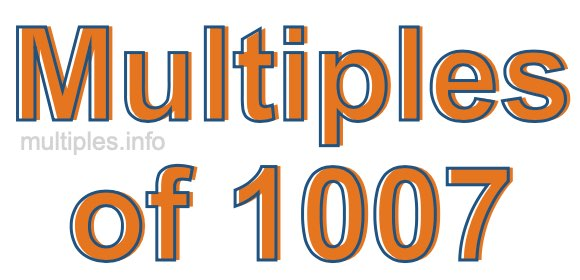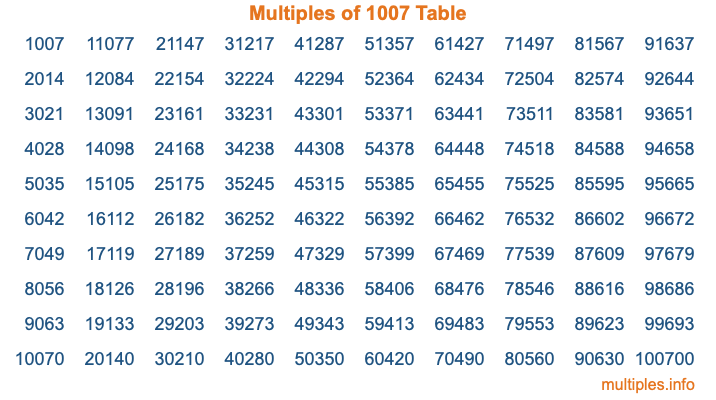Multiples of 1007Welcome to the Multiples of 1007 page. Here we will first teach you everything you will ever need to know about the multiples of 1007, and then give you a study guide summary of everything we taught you to make sure you remember it all. Use this page to look up facts and learn information about the multiples of 1007. This page will make you a multiples of one thousand seven expert!

Definition of Multiples of 1007
Multiples of 1007 are all the numbers that when divided by 1007 equal an integer. Each of the multiples of 1007 are called a multiple. A multiple of 1007 is created by multiplying 1007 by an integer.

Therefore, to create a list of multiples of 1007, you start with 1 multiplied by 1007, then 2 multiplied by 1007, then 3 multiplied by 1007, and so on for as long as you want. Thus, the list of the first five multiples of 1007 is 1007, 2014, 3021, 4028, and 5035. To see a larger list of multiples of 1007, see the printable image of Multiples of 1007 further down on this page. We also have a category where you can choose any nth multiple of 1007.

Multiples of 1007 Checker
The Multiples of 1007 Checker below checks to see if any number of your choice is a multiple of 1007. In other words, it checks to see if there is any number (integer) that when multiplied by 1007 will equal your number. To do that, we divide your number by 1007. If the the quotient is an integer, then your number is a multiple of 1007.

Is  a multiple of 1007?

Least Common Multiple of 1007 and ...
A Least Common Multiple (LCM) is the lowest multiple that two or more numbers have in common. This is also called the smallest common multiple or lowest common multiple and is useful to know when you are adding our subtracting fractions. Enter one or more numbers below (1007 is already entered) to find the LCM.

Check out our LCM Calculator if you need more details about the Least Common Multiple or if you need the LCM for different numbers for adding and subtraction fractions.

nth Multiple of 1007
As we stated above, 1007 is the first multiple of 1007, 2014 is the second multiple of 1007, 3021 is the third multiple of 1007, and so on. Enter a number below to find the nth multiple of 1007.

th multiple of 1007

Multiples of 1007 vs Factors of 1007
1007 is a multiple of 1007 and a factor of 1007, but that is where the similarities end. All postive multiples of 1007 are 1007 or greater than 1007. All positive factors of 1007 are 1007 or less than 1007.

Below is the beginning list of multiples of 1007 and the factors of 1007 so you can compare:

Multiples of 1007: 1007, 2014, 3021, 4028, 5035, etc.

Factors of 1007: 1, 19, 53, 1007

As you can see, the multiples of 1007 are all the numbers that you can divide by 1007 to get a whole number. The factors of 1007, on the other hand, are all the whole numbers that you can multiply by another whole number to get 1007.

It's also interesting to note that if a number (x) is a factor of 1007, then 1007 will also be a multiple of that number (x).

Multiples of 1007 vs Divisors of 1007
The divisors of 1007 are all the integers that 1007 can be divided by evenly. Below is a list of the divisors of 1007.

Divisors of 1007: 1, 19, 53, 1007

The interesting thing to note here is that if you take any multiple of 1007 and divide it by a divisor of 1007, you will see that the quotient is an integer.

Multiples of 1007 Table
Below is an image of the first 100 multiples of 1007 in a table. The table is in chronological order, column by column. The first column has the first ten multiples of 1007, the second column has the next ten multiples of 1007, and so on.The Multiples of 1007 Table is also referred to as the 1007 Times Table or Times Table of 1007. You are welcome to print out our table for your studies.

Negative Multiples of 1007
Although not often discussed or needed in math, it is worth mentioning that you can make a list of negative multiples of 1007 by multiplying 1007 by -1, then by -2, then by -3, and so on, to get the following list of negative multiples of 1007:

-1007, -2014, -3021, -4028, -5035, etc.

Multiples of 1007 Summary
Below is a summary of important Multiples of 1007 facts that we have discussed on this page. To retain the knowledge on this page, we recommend that you read through the summary and explain to yourself or a study partner why they hold true.

There are an infinite number of multiples of 1007.

A multiple of 1007 divided by 1007 will equal a whole number.

1007 divided by a factor of 1007 equals a divisor of 1007.

The nth multiple of 1007 is n times 1007.

The largest factor of 1007 is equal to the first positive multiple of 1007.

1007 is a multiple of every factor of 1007.

1007 is a multiple of 1007.

A multiple of 1007 divided by a divisor of 1007 equals an integer.

1007 divided by a divisor of 1007 equals a factor of 1007.

Any integer times 1007 will equal a multiple of 1007.

Multiples of a Number
Here you can get the multiples of another number, all with the same attention to detail as we did for multiples of 1007 on this page.

Multiples of
Multiples of 1008
Did you find our page about multiples of one thousand seven educational? Do you want more knowledge? Check out the multiples of the next number on our list!